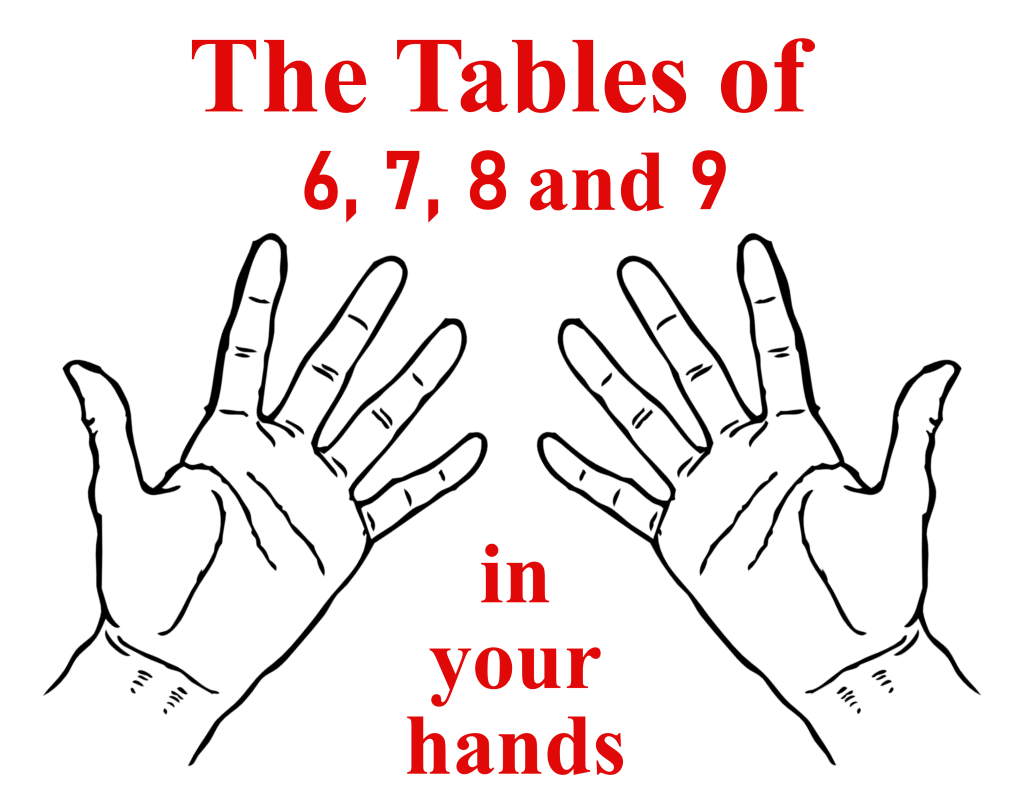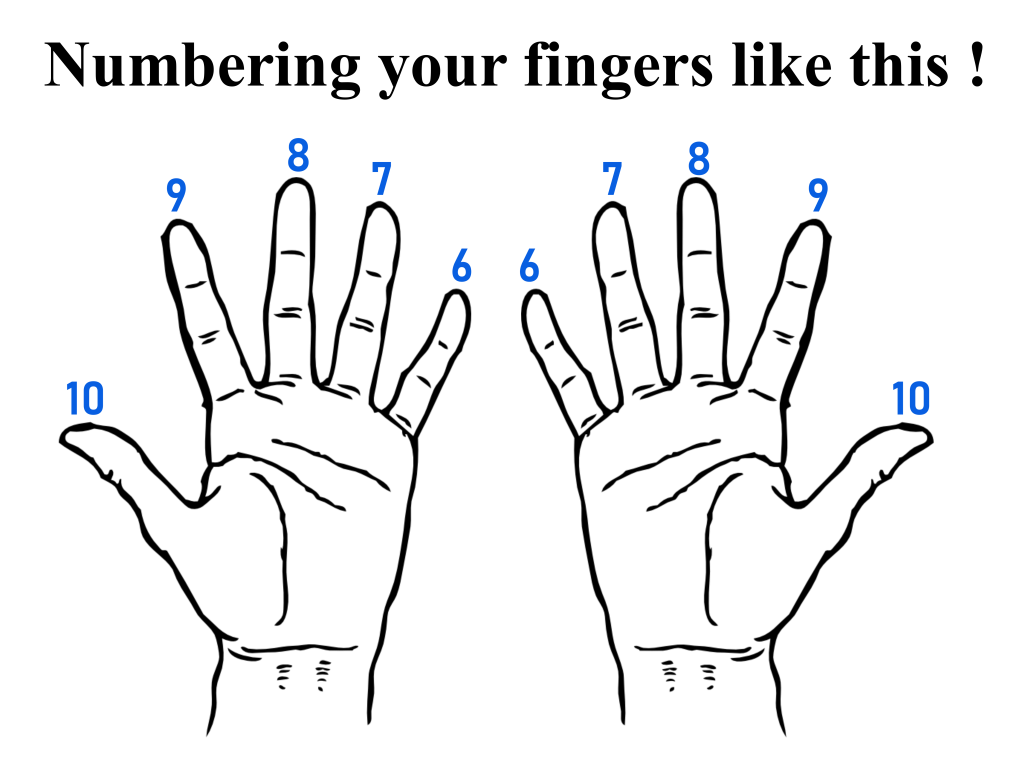Search IntMath
Close

# The Ultimate List of Math Hacks, Tricks, and Tips

By Hugo Pegley, 26 May 2022

No matter your age or grade level, math can be tough at times. Whether you’re learning geometry, division, or fractions, or a parent teaching your kid their times tables, math always seems to find a way to trip students up. Have no fear however, because we have compiled the largest list of math hacks on the Internet. We’ve scoured all the blogs, YouTube videos, textbooks, and more to deliver the 20 most useful math hacks and tricks to help you tackle even the toughest problems and help save you time in math class.

## MULTIPLICATION TRICKS

### Multiplication by 4 made easy

Multiplying by 4 is easy when you know this simple trick!

All you have to do is take the number you are multiplying by 4, and multiply by 2. Then, multiply it by 2 again. That’s it, that’s the math hack!### Multiplying 5 times any even number

Multiplying an even number by 5 is a breeze with this hack!### Multiplying by 6

Want to multiply 6 by an even number? Check out an easy hack to make it simple!

### Multiply numbers by eleven

Eleven can be a tricky number to multiply, but with this math hack you'll be there in no time and will enjoy math class!

### Multiplication From 10-19

Wondering how to simplify multiplication of two numbers both between 10 and 19? Check this out:

### This is how Japanese children learn to multiply!

The Japanese teach their kids to multiply with this math trick in math class!

### Multiplying numbers that end in zero

Learn how to multiply numbers that end in zero

## FRACTIONS TRICKS

### Butterfly method to add and subtract fractions

The butterfly method can simplify tough fraction problems for you. It also looks cool!

### Find fractions of whole numbers with reverse Zorro.

The Reverse Zorro is a unique trick to find whole number fractions quickly. Check it out!

## SQUARING AND CUBING TRICKS

### Quickly square a two-digit number that ends in 5

If you have a two-digit number that ends in 5, you can easily square it with this simple math trick!

### Find the cube root without a calculator

Learn this math trick which helps you find the cube root without a calculator!

##DIVISION TRICKS

These division math hacks will save you time and help you with those tricky division math problems!

##ASSORTED MATH TRICKS

######Quickly and Easily Calculate Percentages

Want to find a percentage quickly? Try this quick math hack!

### Figuring Out An Hourly Rate

Converting from an annual salary to an hourly rate is easy with this simple math hack!

###How to Add Large Numbers Easily!

Sometimes you need to use made up numbers on the SAT or ACT or other tests. Learn how to do this effectively!

## Conclusion

We hope you enjoyed this list of ALL the best math hacks and math tricks to help you get through your schoolwork. Be sure to bookmark this page to come back when you need to find a quicker way to answer the problems!

Be the first to comment below.

### Comment Preview

HTML: You can use simple tags like <b>, <a href="...">, etc.

To enter math, you can can either:

1. Use simple calculator-like input in the following format (surround your math in backticks, or qq on tablet or phone):
a^2 = sqrt(b^2 + c^2)
(See more on ASCIIMath syntax); or
2. Use simple LaTeX in the following format. Surround your math with $$ and $$.
$$\int g dx = \sqrt{\frac{a}{b}}$$
(This is standard simple LaTeX.)

NOTE: You can mix both types of math entry in your comment.